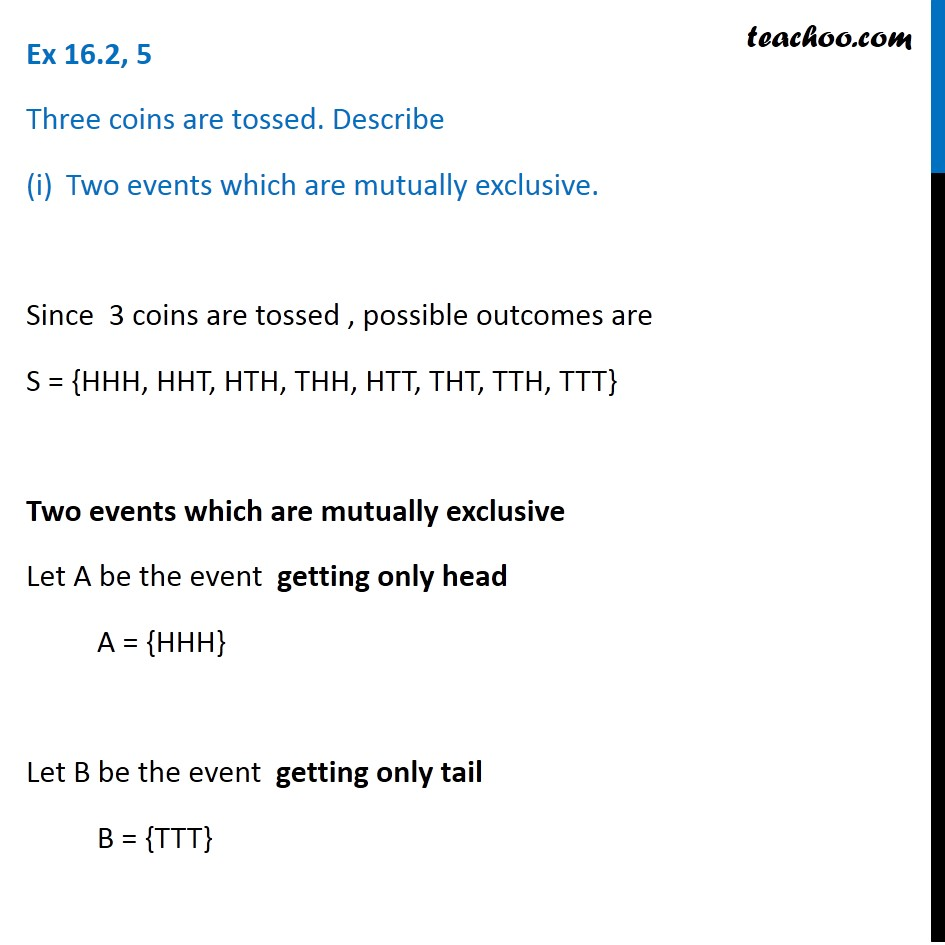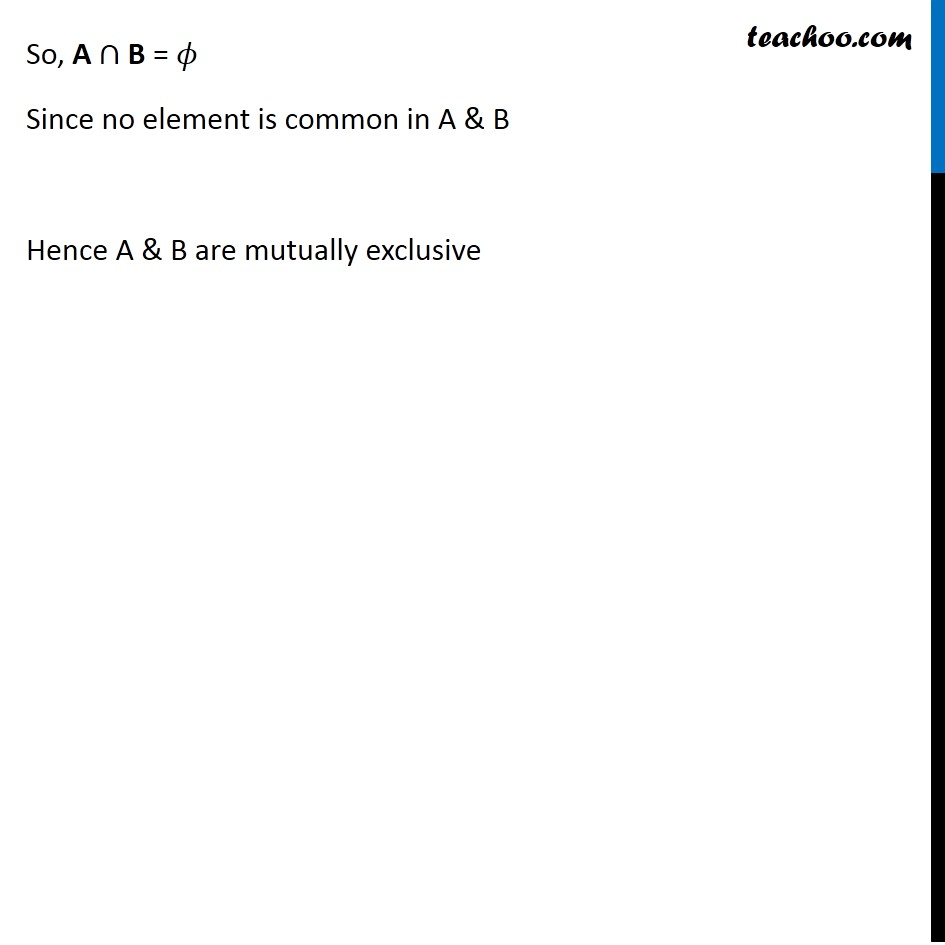1. Chapter 16 Class 11 Probability (Term 2)
2. Concept wise
3. Mutually Exculsive and Exhaustive Events

Transcript

Ex 16.2, 5 Three coins are tossed. Describe Two events which are mutually exclusive. Since 3 coins are tossed , possible outcomes are S = {HHH, HHT, HTH, THH, HTT, THT, TTH, TTT} Two events which are mutually exclusive Let A be the event getting only head A = {HHH} Let B be the event getting only tail B = {TTT} So, A ∩ B = 𝜙 Since no element is common in A & B Hence A & B are mutually exclusive

Mutually Exculsive and Exhaustive Events

Chapter 16 Class 11 Probability (Term 2)
Concept wise